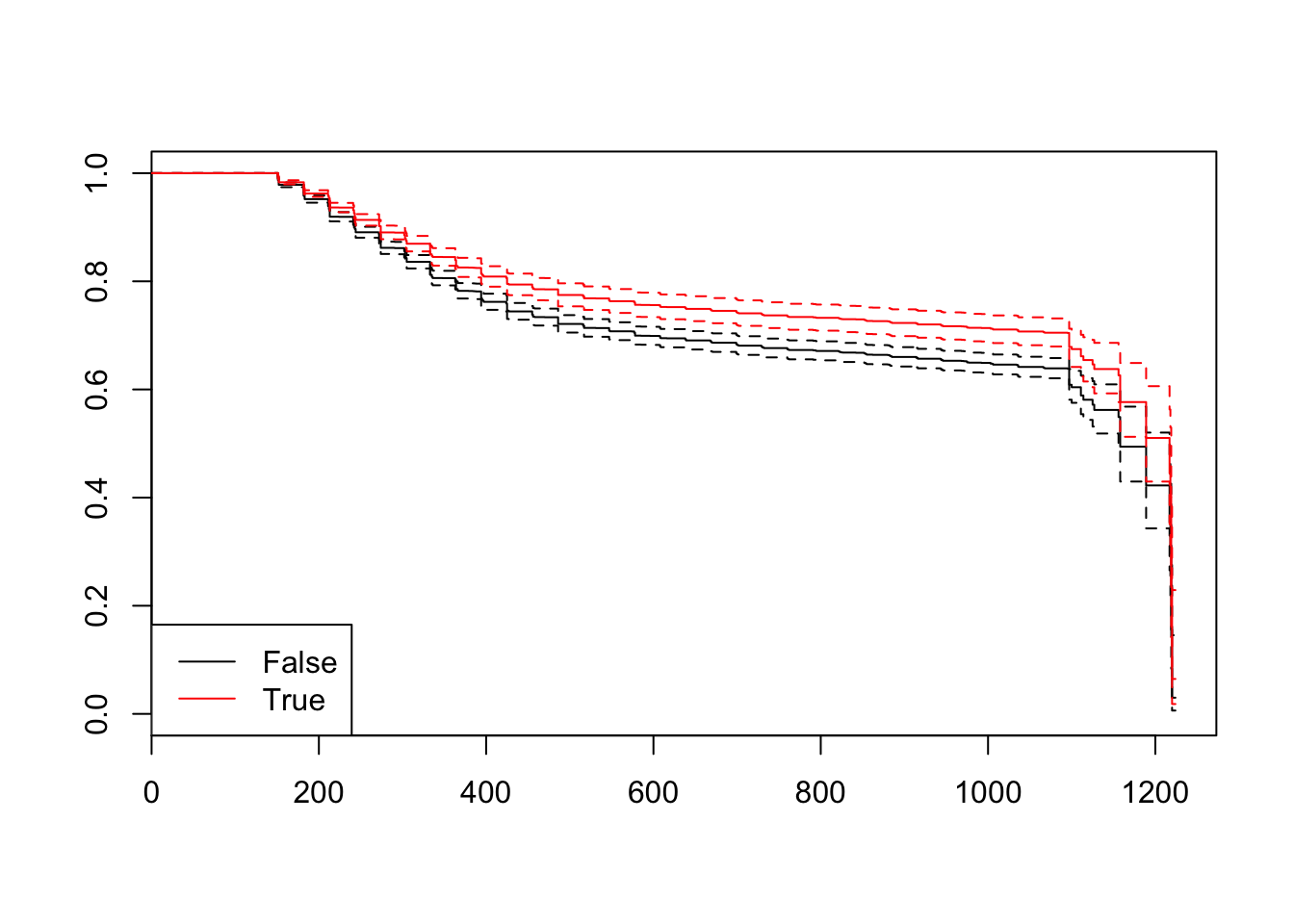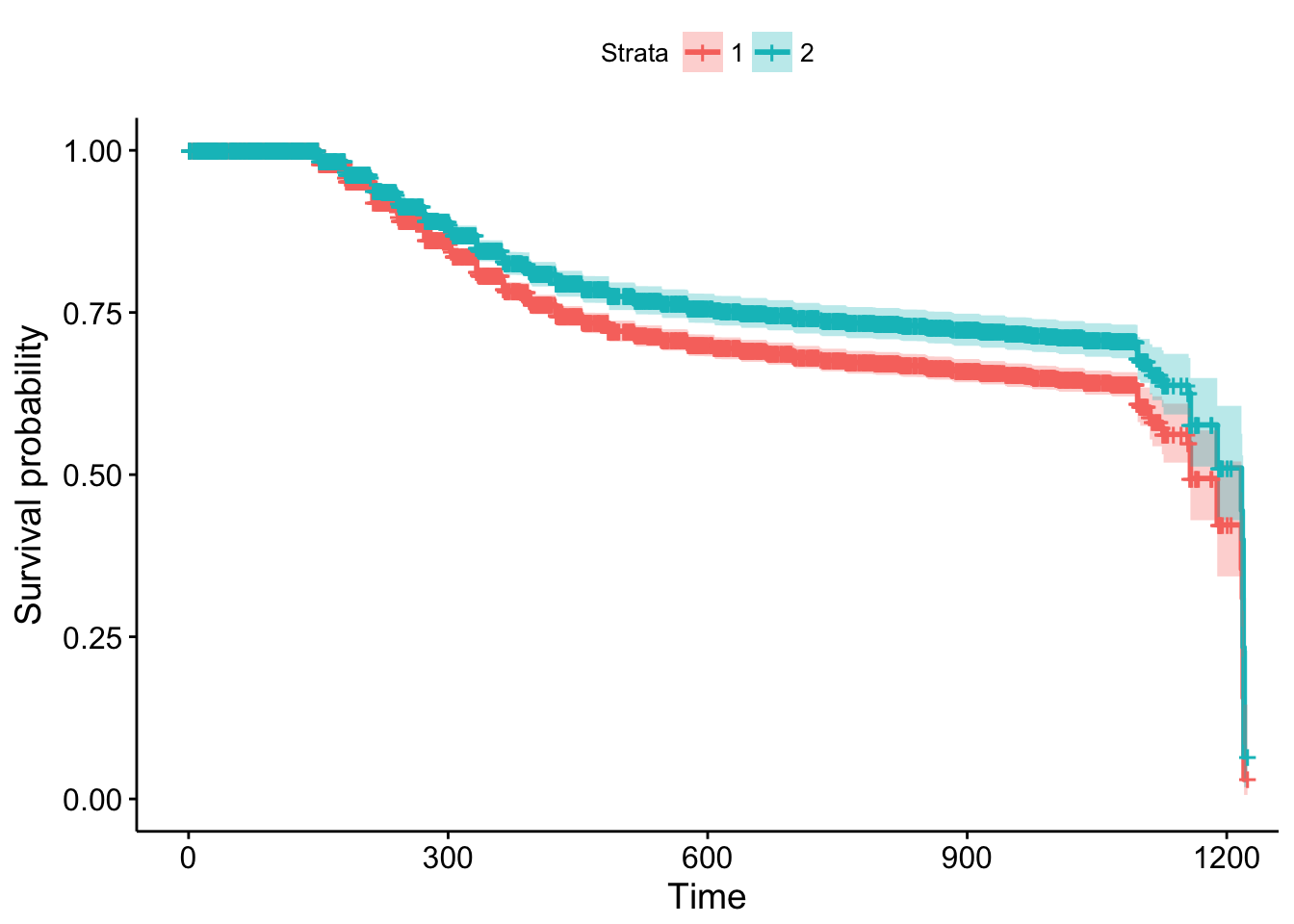From a survival analysis point of view, we want to obtain also estimates for the survival curve. Remember that if we do not use a model, we can apply the Kaplan-Meier estimator. However, when a Cox model is used to fit survival data, survival curves can be obtained adjusted for the explanatory variables used as predictors. These are called adjusted survival curves and, like Kaplan-Meier curves, these are also plotted as step functions.

The hazard formula seen before can be converted to a survival function as

$S(t|\textbf X) = \bigg[ S_0(t) \bigg]^{e^{\sum_{j=1}^p \beta_j X_j}}.$

This survival function formula is the basis for determining adjusted survival curves. The estimates of $$\hat S_0(t)$$ and $$\hat b_j$$ are provided by the computer program that fits the Cox model. The $$X$$’s, however, must first be specified by the investigator before the computer program can compute the estimated survival curve.

Typically, when computing adjusted survival curves, the value chosen for a covariate being adjusted is an average value like an arithmetic mean or a median.

The survfit function estimates $$S(t)$$, by default at the mean values of the covariates:

m2 <- m_red
newdf <- data.frame(IsBorrowerHomeowner = levels(loan_filtered$IsBorrowerHomeowner), LoanOriginalAmount2 = rep(mean(loan_filtered$LoanOriginalAmount2), 2))
fit <- survfit(m2, newdata = newdf)
#summary(fit) # to see the estimated values
plot(fit, conf.int = TRUE, col = c(1,2))
legend("bottomleft", levels(newdf[,1]), col = c(1, 2), lty = c(1,1))# another option using the survminer package
survminer::ggsurvplot(fit, data = newdf)# easier... without refitting
#                    variable = loan_filtered\$IsBorrowerHomeowner)
For some help with the survminer package… Download the cheatsheet here.